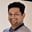Related Tags

java
communitycreator

# How to find the first occurrence using binary search in JavaTarun Telang

## Introduction

A binary search is an algorithm that searches for an element in a sorted array.

In this shot, we will modify the binary search algorithm to return the first occurrence of an integer in an array of integers sorted in ascending order.

## Algorithm

In binary search, once we encounter the search target, we stop searching and return the middle index as the index of the search target. If the search ends with an empty half (left > right), then we terminate the search, returning “element is not found.”

In order to get the first occurrence, even after encountering the search target, we keep continuing the search for the target element on the left half until we reduce our search space to a single element (left = right).

The search is terminated in the following situations:

1. If the target element is not present.
2. Your current search space is reduced to a single element with the first occurrence of the search target.

## Implementation using Java

Below is the Java code to implement the logic described above. You may run this to see it return the first occurrence of the search target for various test cases:

public class Main {
public static int getFirstOccurence(int[] array, int target){

if (array == null || array.length == 0)
return -1;

// Intializing left and right positions
// of the search space
int left = 0;
int right = array.length - 1;

// Searches until you have atleast two elements
while(left < right) {
// Prevents integer overflow
int mid = left + (right - left)/2;

// if middle element is equal to search target,
if(array[mid] == target) {
// discard search space to the right of mid.
right = mid;
} else {
// discard search space from left to mid
left = mid + 1;
}
}

// after while loop, left will be equal to right
// i.e. you have only one element in search space
// else return its index as first index.
return (array[left] == target) ? left : -1;
}

public static void main(String[] args) {
// empty array
int[] test = {};

// array with odd length
int[] test0 = {0, 0, 1, 1, 2, 3};

// array with single element containing search target
int[] test1 = {1};

// array with single element not containing search target
int[] test2 = {0};

// array with even length
int[] test3 = {0, 0, 2, 2, 2, 3};

// array containing search target at the end
int[] test4 = {0, 0, 0, 0, 3};

// array not containing search target
int[] test5 = {0, 1, 1, 2, 2};

System.out.println(getFirstOccurence(test, 1));
System.out.println(getFirstOccurence(test0, 1));
System.out.println(getFirstOccurence(test1, 1));
System.out.println(getFirstOccurence(test2, 2));
System.out.println(getFirstOccurence(test3, 2));
System.out.println(getFirstOccurence(test4, 3));
System.out.println(getFirstOccurence(test5, 3));
}
}

## Next Steps

To further strengthen your understanding, try implementing the code to return the last occurrence of the target element using a similar approach.

RELATED TAGS

java
communitycreator

CONTRIBUTORTarun Telang
RELATED COURSES

View all Courses

Keep Exploring

Learn in-demand tech skills in half the time